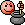# For Sale:Nikko stirling targetmaster 6-24x56

Price drop

#### bobjs

##### Well-Known Member
Nikko stirling targetmaster 6-24x56

30mm

mildot ret <<<<<<<<<<<<<<<<<<<<<<<<<<<<<<<<<<<<<<<<<<<good condition

£160inc postage

View attachment 73232View attachment 73233View attachment 73234
<<<<<<<<<<<<<<<<<theres a clue#### Ah8217bris

##### Well-Known Member
<<<<<<<<<<<<<<<<<theres a clueDont forget the box label too Bob